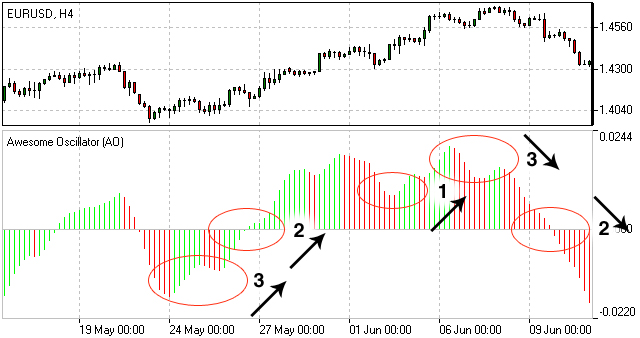# How to Use Awesome Oscillator Indicator (AO)

### Awesome Oscillator Definition

Awesome Oscillator (AO) is a momentum indicator reflecting the precise changes in the market driving force which helps to identify the trend’s strength up to the points of formation and reversal.

### How to Use Awesome Oscillator Indicator in Forex Trading

There are three main signals which may be seen:

1. Saucer – three consecutive columns above the nought line the first two of which must be colored red (the second one is lower than the first one) while the third one is colored green and higher than the previous (second) one. Such a formation would be a clear Buy signal whilst inverted and vertically flipped formation would serve as a Sell signal.
2. Nought line crossing – the histogram crosses the naught line in an upward direction changing its values from negative to that of positive ones. In this situation we have a Buy signal. The Sell signal would be a reversed pattern.
3. Two pikes – the indicator displays a Buy signal when the figure is formed by two consecutive pikes both of which are below the naught line and the later-formed pike is closer to the zero level than the earlier-formed one. The Sell signal would be given by the reverse formation.Awesome Oscillator Strategy includes 3 ways of trading. The first way is to open a sell position when the oscillator is below the zero line forming a peak, and open a buy position when the oscillator is above the zero line forming a gap.

Another way is to open a sell position when the oscillator forms two peaks above the zero line, where the second high is lower than the previous one. And, conversely, traders watch to open a buy position when the oscillator forms to lows below the zero line with the last one not as low as the previous one.

The third way is to account crossing the zero line. When the oscillator crosses it from up to down, it is time to open a sell position and when it crosses from down to up, it is time to open a buy position.

### Awesome Oscillator Formula (Calculation)

Awesome Oscillator is a 34-period simple moving average, plotted through the central points of the bars (H+L)/2, and subtracted from the 5-period simple moving average, graphed across the central points of the bars (H+L)/2.

MEDIAN PRICE = (HIGH+LOW)/2

AO = SMA(MEDIAN PRICE, 5)-SMA(MEDIAN PRICE, 34)

where

SMA — Simple Moving Average.

Tentang Penulis#### Syaifullah Chattar

CEO & Founder OmahForex, start trading forex since 2005 and is currently as a full-time trader.